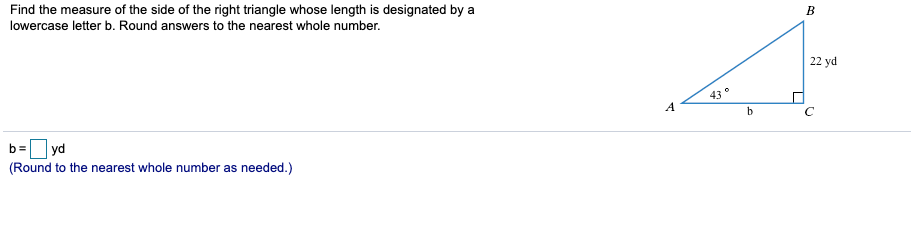# Find the measure of the side of the right triangle whose length is designated bylowercase letter b. Round answers to the nearest whole number.a22 yd43AhCb=yd(Round to the nearest whole number as needed.)

Question
106 views

i have attached the screen shot, i am having trouble solving for b.

I have gotten to this step

tan43=22/bhelp_outlineImage TranscriptioncloseFind the measure of the side of the right triangle whose length is designated by lowercase letter b. Round answers to the nearest whole number. a 22 yd 43 A h C b= yd (Round to the nearest whole number as needed.) fullscreen
check_circle

Step 1

Refer to the question , we have to find the side b of the triangle.

Use the tangent formula of trigonometry as,

Step 2

Then use the above relation to find the value of b. As the length opposite to the angle is perpendicular and base is where the angle lies.

Step 3

Now to find b cross ...

### Want to see the full answer?

See Solution

#### Want to see this answer and more?

Solutions are written by subject experts who are available 24/7. Questions are typically answered within 1 hour.*

See Solution
*Response times may vary by subject and question.
Tagged in

### Trigonometric Ratios